# Fields and Waves Lesson 5 1 TIMEVARYING FIELDS

• Slides: 8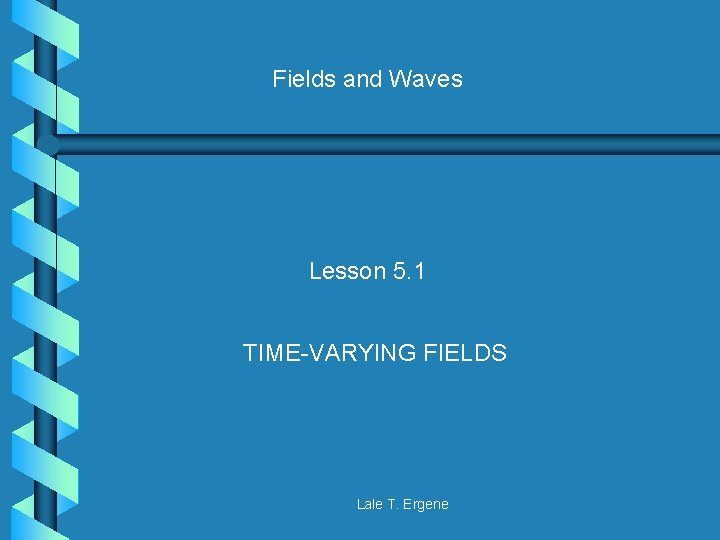Fields and Waves Lesson 5. 1 TIME-VARYING FIELDS Lale T. Ergene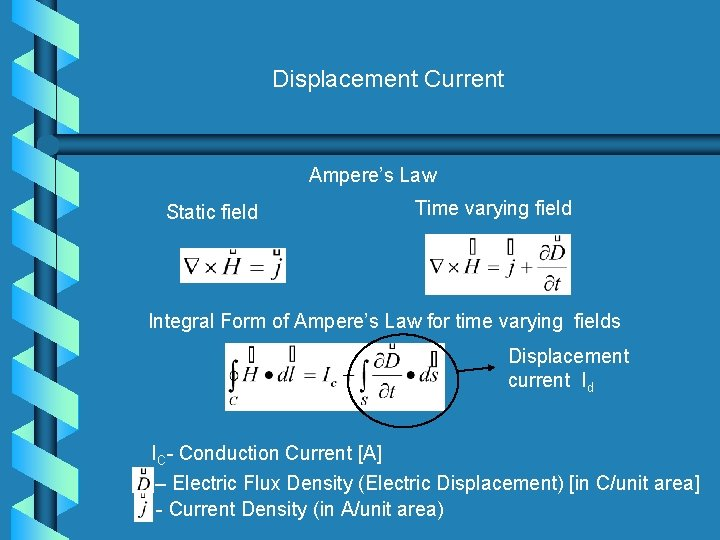Displacement Current Ampere’s Law Static field Time varying field Integral Form of Ampere’s Law for time varying fields Displacement current Id IC- Conduction Current [A] – Electric Flux Density (Electric Displacement) [in C/unit area] - Current Density (in A/unit area)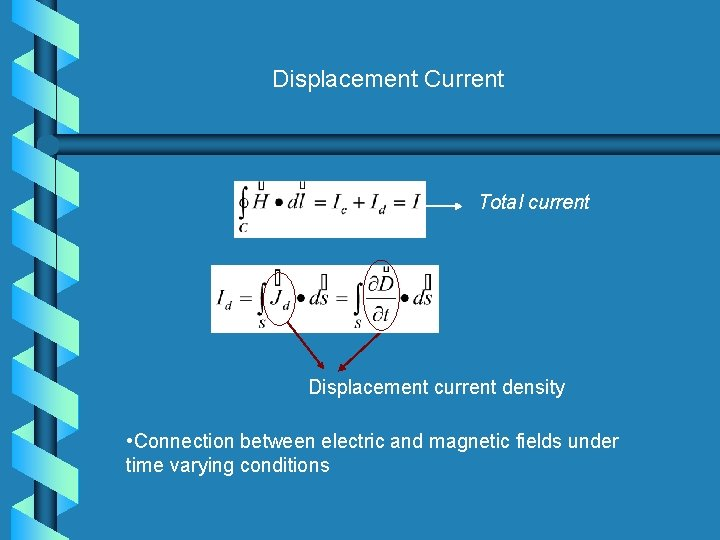Displacement Current Total current Displacement current density • Connection between electric and magnetic fields under time varying conditions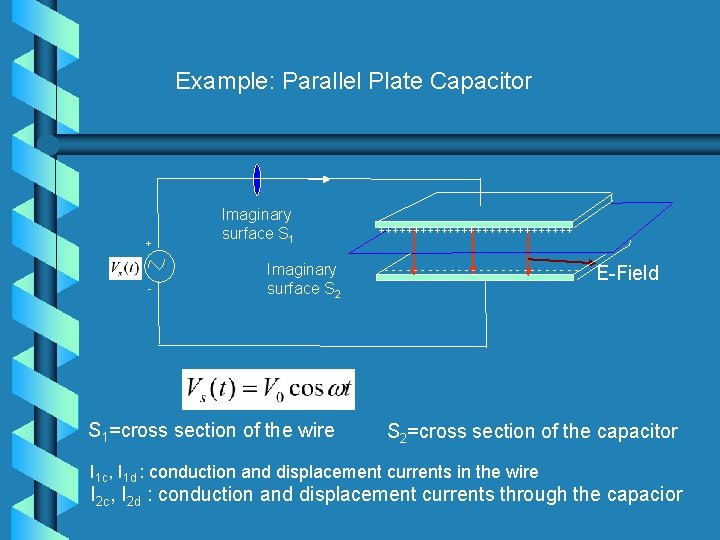Example: Parallel Plate Capacitor + - Imaginary surface S 1 Imaginary surface S 2 S 1=cross section of the wire ++++++++++++++ -------------- E-Field S 2=cross section of the capacitor I 1 c, I 1 d : conduction and displacement currents in the wire I 2 c, I 2 d : conduction and displacement currents through the capaciorExample: Parallel Plate Capacitor In a perfect conductor From the circuit theory: Total current in the wire: I 1 d=0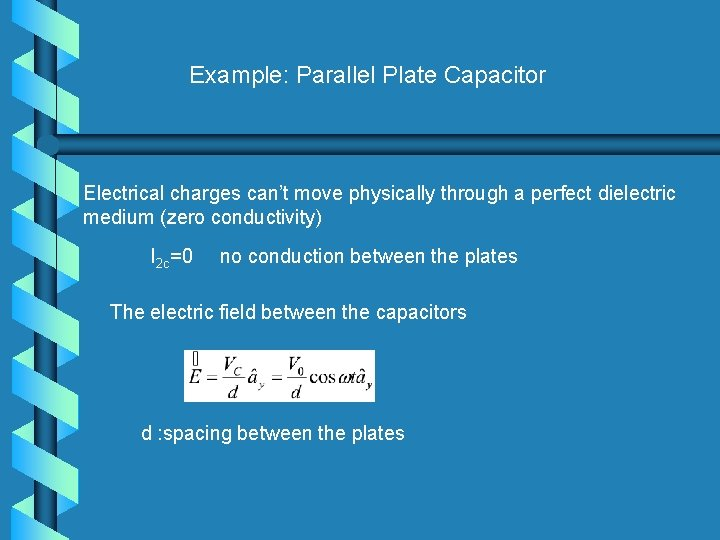Example: Parallel Plate Capacitor Electrical charges can’t move physically through a perfect dielectric medium (zero conductivity) I 2 c=0 no conduction between the plates The electric field between the capacitors d : spacing between the plates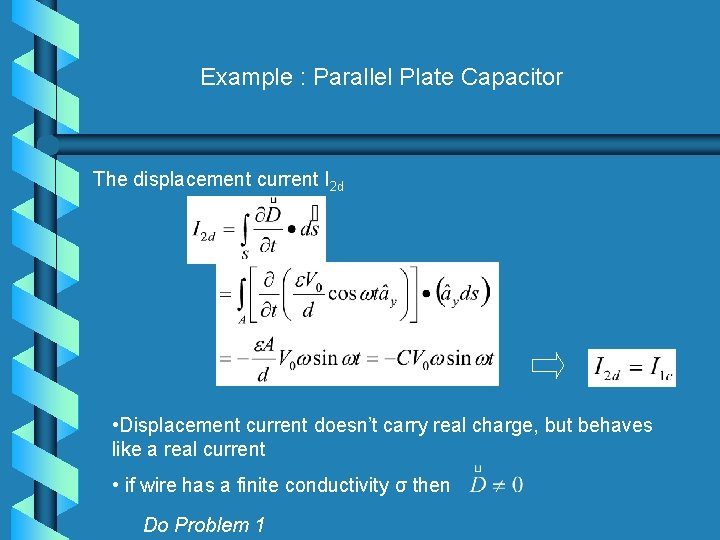Example : Parallel Plate Capacitor The displacement current I 2 d • Displacement current doesn’t carry real charge, but behaves like a real current • if wire has a finite conductivity σ then Do Problem 1Boundary Conditions • Boundary conditions derived for electrostatics and magnetostatics remain valid for time-varying fields: dielectric-dielectric boundary dielectric-conductor boundary